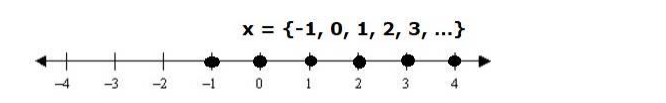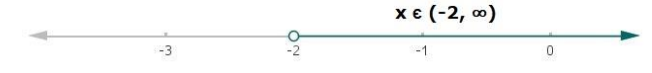# Solve each of the following in equations and represent the solution set on

Question:

Solve each of the following in equations and represent the solution set on the number line.

$3 x+8>2$, where (i) $x \in Z$, (ii) $x \in R$.

Solution:

(i) $3 x+8>2, x \in Z$

Subtracting 8 from both the sides in above equation

$3 x+8-8>2-8$

$3 x>-6$

Dividing both the sides by 3 in above equation

$\frac{3 x}{3}>\frac{-6}{3}$

Thus, $x>-2$

Since x is an integer

Therefore, possible values of x can be

$x=\{-1,0,1,2,3, \ldots\}$(ii) $3 x+8>2, x \in R$

Subtracting 8 from both the sides in above equation

$3 x+8-8>2-8$

$3 x>-6$

Dividing both the sides by 3 in above equation

$\frac{3 x}{3}>\frac{-6}{3}$

Thus, $x>-2$

$x \in(-2, \infty)$